### TESTFUNC Statement

TESTFUNC functions ;

where functions are either parameters in the model or parametric functions computed in the SAS programming statements.

When the estimates in a model are asymptotically multivariate-normal, any continuous and differentiable function of the estimates is also normally distributed. In the TESTFUNC statement, you can test these parametric functions using z-tests. The form of the null hypothesis is as follows::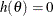whereis the set of model parameters (independent or dependent) in the analysis and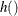is a continuous and differentiable function of the model parameters.

For example, if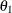,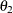, and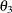are parameters in the model, and you want to test whetherandare the same and whetheris the same as the average ofand, you can use the following statements:

testfunc   t1_t2_diff t3_t1t2_diff;
t1_t2_diff   = theta1 - theta2;
t3_t1t2_diff = theta3 - (theta1 + theta2)/2;


In the TESTFUNC statement, you test two functions: t1_t2_diff and t3_t1t2_diff. These two functions are defined in the SAS programming statements that follow after the TESTFUNC statement. Thus, t1_t2_diff represents the difference betweenand, and t3_t1t2_diff represents the difference betweenand the average ofand.

See the SIMTESTS statement if you want to test several null hypotheses simultaneously.